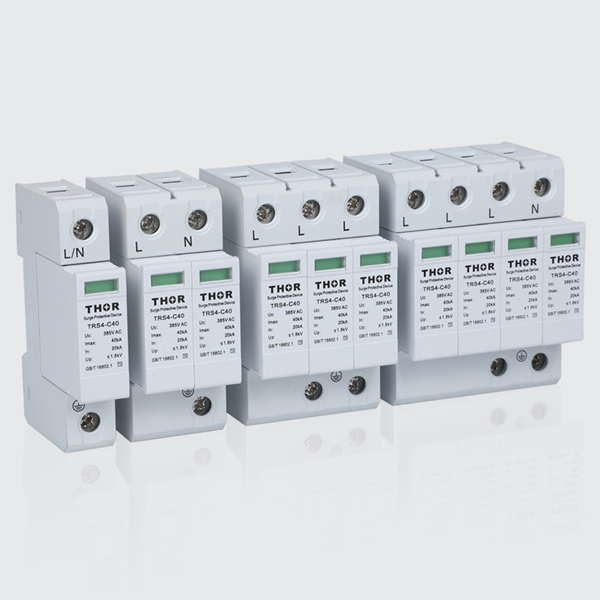## The meaning of Uc on SPD

Data：2021-12-20The meaning of Uc on SPD

Each power SPD is marked with a Uc=xxxV, we must understand the meaning of Uc when selecting SPD products, the relationship between Uc and SPD using power system Un (rated voltage), Uc and SPD product selection of varistor operating voltage Relationship.

1.The meaning of Uc:

GB 18802.1-2011 Low Voltage Surge Protector (SPD) Part 1: The definition of performance requirements and test methods for low-voltage power distribution systems. Uc of SPD refers to the maximum continuous working voltage of SPD, which can be continuously applied to SPD protection The maximum effective value of AC voltage in the mode.

The definition contains 3 key elements:

1) Can be continuously applied to SPD protection mode

Long-term uninterrupted voltage between SPD terminals.

2) Maximum AC voltage

Including the overvoltage for a period of time due to power failure during the use of the SPD.

According to the power system overvoltage analysis, when a single-phase ground fault occurs in a three-phase power supply, the voltage between the LN of the faulty phase is 0, and the voltage between the LNs of the remaining two phases is 3 times the normal phase voltage, so when When the phase voltage of the three-phase power system is 220V, the Uc of the SPD should be 380V.

1) Valid value

For SPD, the Uc value is the effective value rather than the peak value.

1. The relationship between Uc and SPD power supply system Un (rated voltage)

The AC rated voltage Un used by SPD is generally expressed as 0.4kV or 220/380V, meaning that the normal working voltage between the power system line (LL) is 380V, and the normal working voltage between the line zero (LN) is 220V. Therefore, according to SPD Usage conditions and standard requirements for Uc, the corresponding requirements for Un and Uc are:

Three-phase AC system Uc =3 Un

DC system Uc=1.2Un

1. The relationship between Uc and the operating voltage of the varistor selected for SPD products

The varistor for SPD has the characteristic of converting the internal resistance from high resistance to low resistance when the applied voltage reaches a certain value. The operating voltage is defined as the DC voltage applied, and the DC voltage when the varistor leakage current is 1mA value.

For the SPD used in the three-phase AC power system, Uc is the effective value, and the operating voltage of the varistor can be understood as the peak value of the AC voltage.

When Uc is set to 385V, the operating voltage value of the varistor should be 2 times Uc. Considering that the deviation range of the varistor resistance voltage is 10%, the coefficient should be increased by 1.1. Therefore, the operating voltage value of the varistor is not lower than U=1.1*2*Uc=1.1*1.414*385=599V